# Volumetric heat diffusion skinning

November 19th, 2009

After seeing our rigging tutorial, a number of you asked me how we calculate our vertex weights. However, many of you reading the blog are probably asking yourselves a more basic question:

### What are vertex weights?

When we first load the rabbit, it's a static model: just a collection of triangles with an image wrapped around it. Next, we create the skeleton, which is a set of line segments (bones). Finally, we get to the tricky part. How do we attach the model to the skeleton so that they both move in the same way?

For every vertex (triangle point) in the model, we have to figure out which bones it should be attached to, and how strong each attachment should be. This information is stored in the vertex weighting. Below you can see the static model, the model with a skeleton, and the area of influence for each bone.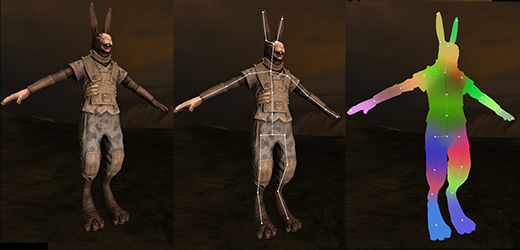Using the vertex weights, we can match the model's pose to the skeleton's. Whenever a bone moves, we can apply the bone's movement to all vertices that are attached to it, modulated by the attachment strength. The attachment strength is important for smooth deformation at joints, where vertices are attached to multiple bones. Here is an example of pose matching using vertex weights:Usually these weights are painstakingly applied by hand, using a virtual 'paintbrush' or some kind of 'envelope' system. However, this is a long, tedious process, and I didn't want to force Aubrey (and modders) to go through it if it could be automated.

### How do we calculate them automatically?

The most obvious way to calculate vertex weights is to attach each vertex to the closest bones, weighted by distance. At first glance the results look ok, but if you move the bones around, you start to see problems. For example, the bones in one foot can affect vertices in the other because they're in close proximity, even though they're not connected.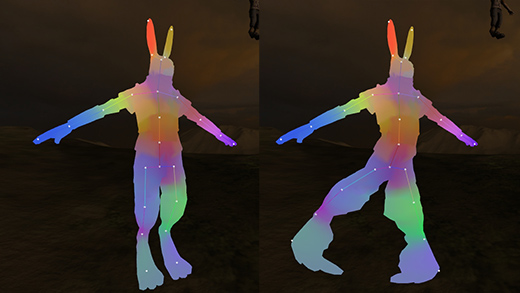Clearly we're can't just use simple Cartesian distance -- we need the distance of the shortest path within the volume of the model. For example, the distance from the right foot to the left foot should be about equal to the distance from the right foot to the right shoulder, because the path must go up one leg and down the other. When working with volumetric calculations, it's often helpful to voxellize the model. Voxellization turns the model into a 3D cube structure that approximates the model's shape.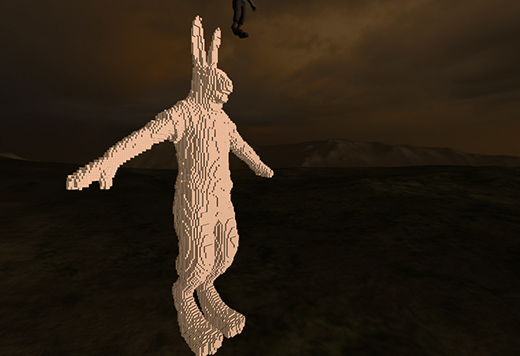I found that the easiest way to think about this was in terms of heat. We can treat each bone as a heating filament, and then diffuse the heat through the voxel model. Diffusion is pretty easy using a voxel model -- we can just set each voxel's heat to a weighted average of its neighbors' heat, and repeat as necessary until it diffuses completely.

The final heat distribution is proportional to the shortest path from the bone to any point in the model, so we can get the final vertex weights by just comparing the heat from each bone at each vertex. Here is a picture of the foot bone's 'filament', the foot's heat distribution, and its final vertex weights: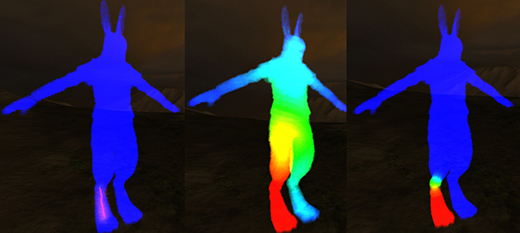I found this algorithm to be intuitive and easy to use, and it eliminates the most tedious part of character modeling. I am very happy with this technique! Confusingly, there's an existing skinning algorithm called 'bone heat', but it has a very confusing implementation, and incorrectly uses surface diffusion instead of volumetric. If I had to name my techique I would call it "volumetric heat diffusion". Here is the rabbit posed in various ways using automatic vertex weights: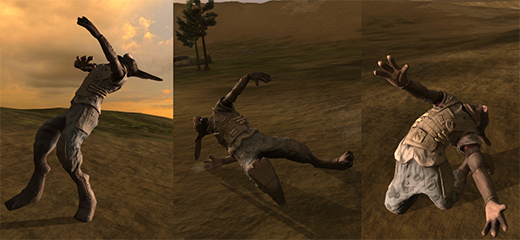There are a couple of ways I can think of to improve this technique, but haven't implemented them yet because they're not urgent. First, the heat diffusion is a massively parallel task, so it could be sped up by orders of magnitude using the GPU. Second, it would be useful to have some interface to make the joint blending harder or softer in specific areas by using different power functions on the heat values.

Do you have any more questions about the vertex weights, or ideas to improve it?